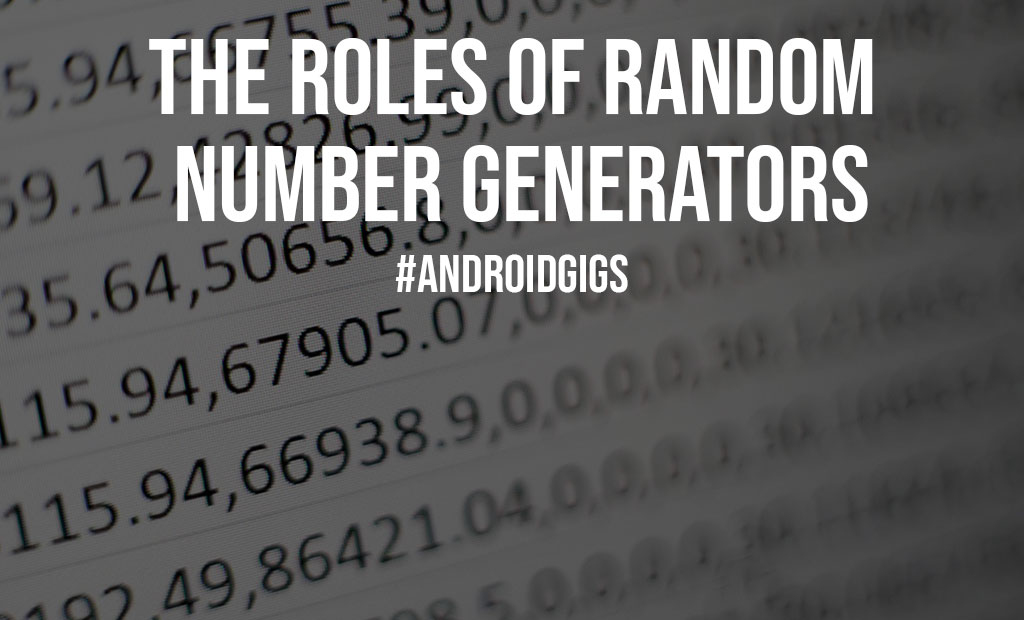# The Roles of Random Number Generators

Random number generators are mathematical constructs, created computationally or through a hardware device, which generates random number sets that display no distinguishable pattern in their distribution or appearance, creating the essence of their name. Games or other software applications that incorporate chance often use these functions or blocks of code.

The random number generator is simply the modern use of instruments that have been in existence forever like the shuffled cards, dice, drawing straws, and flipping coins. Random number generator in computing is implemented by programming algorithms that are determined by deterministic documenting but don’t translate to true randomness since all seed values can be predicted when people know the input values.It is, however, sufficient for most tasks in practice. Authentic randomness isn’t always required; in fact, many software doesn’t take advantage of it at all.

## Ways of creating random numbers

### True Random Numbers

There are two main ways of creating random numbers. The first way is the physical method which generates the randomness from a physical incident that happens randomly. Such an occurrence happens outside the computer.

Measuring and adjusting the results for biases that may exist due to the measurement method. Among these incidents are radiation, photoelectric effects, cosmic background, radioactive decay, atmospheric noise, and other incidents.

Therefore, a number generated from such randomness is said to be a truly random number. As far as hardware is concerned, it is made up of a device that converts energy from one form into another. There are several casinos with this technology, including NetBet. If you wish to casino online, you can do so by visiting their site.

Also Read: Hybris Software Development Outsourcing: What You Need To Know

### Pseudorandom Numbers

The second method of generating random numbers is the computational algorithms which can produce seemingly random results instead of true random numbers. How are they random if they’re determined by a computer?

Because the final results are determined by the seed value or key which is known as an initial value. So, if you know the key value and how the algorithm works, you can reproduce these random numbers. This type of random number generator is often referred to as a pseudorandom number generator since it generates pseudorandom numbers.

Let’s examine some parts of true random number generators popularly called TRNGs and pseudorandom number generators popularly called PRNGs. TRNGs are slower than PRNGs. Their deterministic nature makes them useful when you need to replay a sequence of random events. This can prove extremely useful in code testing, for instance.

TRNGs, however, do not have periodicity and are more suitable for security-sensitive roles such as encryption. A period is how many iterations a PRNG goes through before it repeats itself. In other words, a PRNG with a longer period would require more processing power to speculate and break.

### Algorithm Example for Pseudorandom Number Generator

Computer programs execute code according to a set of rules that needs to be followed. The rules for PRNGs generally revolve around the following:

Accept a seed or key as an input number. Using that seed, perform a series of mathematical operations to produce a result. That number is your random number.

Next, use that resulting random number as the seed at your next iteration

Do this repeatedly to simulate randomness.

If a TRNG relies on computational algorithms and mathematical equations to come up with a random number, then the number is not random. True randomness is achieved by gathering entropy from a natural habitat, such as quantum and electromagnetic phenomena and thermal and atmospheric noise, as well as others.

In one example of random number generating, an instrument that measures radio noise extracts the worth and hands it to the player. Other entropy sources include subatomic particles like radioactive decay.